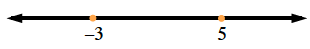### Home > PC > Chapter 7 > Lesson 7.3.3 > Problem7-128

7-128.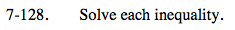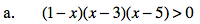The > sign means you need 3 factors when multiplied are positive.
Check values in the intervals about the zeros to find these positive intervals.
Write the answer in interval notation.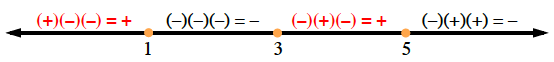(−∞,1) ∪ (3,5)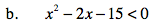1. Factor and find the zeros.
2. Use the line below to test your intervals.
3. How many factors do you have?
4. Should they multiply to a positive or negative number?